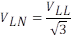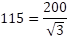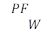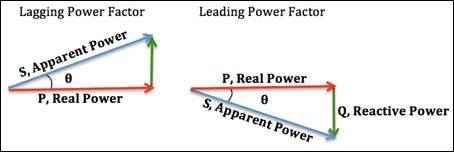# AC and Three Phase Power Cheat Sheet

AC voltage is sometimes expressed like 115/200 volts.  This means 115VLN a.k.a. 200VLL.

VLN = Line to Neutral Voltage (usually rms)

VLL = Line to Line Voltage (usually rms)Power Factor (PF) = The ratio of real power in watts (W) to apparent power in volt amps (VA).  A resistive load has a power factor of 1.  A capacitive load is leading (current leads voltage).  An inductive load is lagging (current lags voltage).Figure 1:  Credit Wikipedia

Example: Calculate phase current given VLL and load power.  To solve, the voltage is converted to Line to Neutral and the power is split in equal thirds.